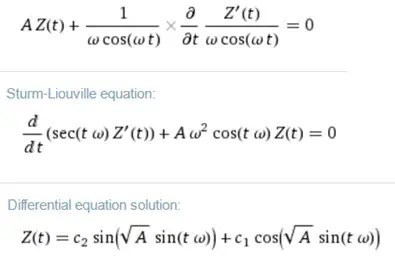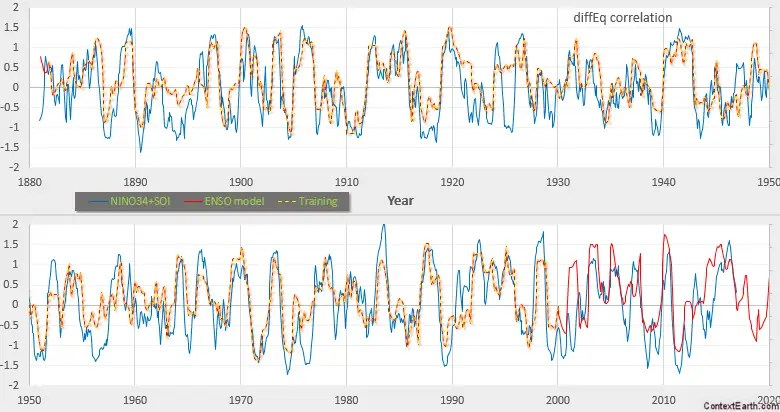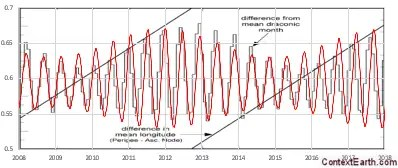# Derivation of an ENSO model using Laplace’s Tidal Equations

[mathjax]Laplace developed his namesake tidal equations to mathematically explain the behavior of tides by applying straightforward Newtonian physics. In their expanded form, known as the primitive equations, Laplace’s starting formulation is used as the basis of almost all detailed climate models. Since that’s what they are designed to do, this post provides the details for solving Laplace’s tidal equations in the context of the El Nino Southern Oscillation (ENSO) of the equatorial Pacific ocean. The derivation and results shown below essentially describe the framework of my presentation at this month’s AGU meeting: Biennial-Aligned Lunisolar-Forcing of ENSO: Implications for Simplified Climate Models

The concise derivation for a model of ENSO depends on reducing Laplace’s tidal equations along the equator. I could not find anyone taking a similar approach anywhere in the literature, even though it appears to be routinely obvious: (1) solve Laplace’s tidal equations in a simplified context, then (2) apply the known tidal forcing and observe if the result correlates or matches the ENSO time series. In fact, it does, as I have shown before (and for QBO as well); but this is the first time that I have worked out the details in full for ENSO. Below is a two part solution.

## Part 1 : Deriving a closed-form solution

For a fluid sheet of average thickness $$D$$, the vertical tidal elevation $$zeta$$, as well as the horizontal velocity components $$u$$ and $$v$$ (in the latitude $$varphi$$ and longitude $$lambda$$ directions).

This is the set of Laplace’s tidal equations (wikipedia). Along the equator, for $$varphi$$ at zero we can reduce this.{begin{aligned}{frac {partial zeta }{partial t}}+{frac {1}{acos(varphi )}}left[{frac {partial }{partial lambda }}(uD)+{frac {partial }{partial varphi }}left(vDcos(varphi )right)right]=0,\{frac {partial u}{partial t}}-vleft(2Omega sin(varphi )right)+{frac {1}{acos(varphi )}}{frac {partial }{partial lambda }}left(gzeta +Uright)=0,\{frac {partial v}{partial t}}+uleft(2Omega sin(varphi )right)+{frac {1}{a}}{frac {partial }{partial varphi }}left(gzeta +Uright)=0,end{aligned}}

where $$Omega$$ is the angular frequency of the planet’s rotation, $$g$$ is the planet’s gravitational acceleration at the mean ocean surface, $$a$$ is the planetary radius, and $$U$$ is the external gravitational tidal-forcing potential.

The main candidates for removal due to the small-angle approximation along the equator are the second terms in the second and third equation. The plan is to then substitute the isolated $$u$$ and $$v$$ terms into the first equation, after taking another derivative of that equation with respect to $$t$$.{begin{aligned}{frac {partial zeta }{partial t}}+{frac {1}{a}}left[{frac {partial }{partial lambda }}(uD)+{frac {partial }{partial varphi }}left(vDright)right]=0,\{frac {partial u}{partial t}}+{frac {1}{a}}{frac {partial }{partial lambda }}left(gzeta +Uright)=0,\{frac {partial v}{partial t}}+{frac {1}{a}}{frac {partial }{partial varphi }}left(gzeta +Uright)=0,end{aligned}}

Taking another derivative of the first equation:{begin{aligned}{afrac {partial^2 zeta }{partial t^2}}&+ frac {partial }{partial t} left[{frac {partial }{partial lambda }}(uD)+{frac {partial }{partial varphi }}left(vDright)right]=0,end{aligned}}

Next, on the bracketed pair we invert the order of derivatives (and pull out the constant $$D$$){begin{aligned}{afrac {partial^2 zeta }{partial t^2}}&+ D left[{frac {partial }{partial lambda }}( frac {partial u }{partial t} )+{frac {partial }{partial varphi }}(frac {partial v }{partial t} )right]=0,end{aligned}}

Notice now that the bracketed terms can be replaced by the 2nd and 3rd of Laplace’s equations{begin{aligned}{frac {partial u}{partial t}}&=-{frac {1}{a}}{frac {partial }{partial lambda }}left(gzeta +Uright)end{aligned}}{begin{aligned}{frac {partial v}{partial t}}&=-{frac {1}{a}}{frac {partial }{partial varphi }}left(gzeta +Uright)end{aligned}}

as{begin{aligned}{a^2frac {partial^2 zeta }{partial t^2}}&- D left[{frac {partial }{partial lambda }}( {{frac {partial }{partial lambda }}left(gzeta +Uright)} )+{frac {partial }{partial varphi }}({{frac {partial }{partial varphi }}left(gzeta +Uright)})right]=0end{aligned}}

The $$lambda$$ terms are in longitude so that we can use a separation of variables approach and create a spatial standing wave for ENSO (i.e. the sloshing model), $$SW(s)$$ where $$s$$ is a wavenumber.{begin{aligned}{frac {partial^2 zeta }{partial t^2}}&- D left[( SW(s) zeta )+{frac {partial }{partial varphi }}({{frac {partial }{partial varphi }}left(gzeta +Uright)})right]=0end{aligned}}That is essentially close to a variation of a Sturm-Liouville equation (see right). To solve this for a plausible set of boundary conditions, we make an adjoint connection between a change in latitudinal forcing with a temporal change:begin{aligned} {frac {partial zeta }{partial varphi } = frac {partial zeta }{partial t} frac {partial t }{partial varphi } } end{aligned}

After properly applying the chain rule, this reduces the equation to a function of $$zeta(t)$$ and $$varphi(t)$$, along with a constant A. The A subsumes the wavenumber SW(s) portion so there will be multiple solutions for the various standing waves, which will be used in fitting the model to the data.$A zeta(t) + frac {1}{ frac {partial varphi }{partial t }} cdot frac {partial}{partial t}frac {zeta'(t)}{ frac {partial varphi }{partial t }} = 0$

So if we fix $$varphi(t)$$ to a periodic function with a long-term mean of zerobegin{aligned} frac {partial varphi }{partial t } = sum_{i=1}^{i=N} k_i omega_i cos(omega_i t) end{aligned}

to describe the tractive latitudinal displacement terms near the equator, then the solution is the following:begin{aligned} zeta(t) = sin( sqrt{A} sum_{i=1}^{i=N} k_i sin(omega_i t) + theta_0 ) end{aligned}

where $$A$$ is an aggregate of the constants of the differential equation and $$theta_0$$ represents the fixed phase offset necessary for aligning on a seasonal peak. This approximation of a tangential tractive force as a cyclic displacement for $$varphi(t)$$ is a subtle yet very effective means of eliminating a big unknown in the dynamics ( this is essentially similar to a Berry phase applied as a cyclic adiabatic process, with the phase being the internal sin modulation ). Not including this approximation leaves us with an indeterminate set of equations.

Now consider that the ENSO itself is precisely the $$frac {partial v}{partial t}$$ term – the horizontal longitudinal acceleration of the fluid, i.e. leading to the observed sloshing in the thermocline – which can be derived from the above by applying the solution to Laplace’s third tidal equation in simplified form above:$frac {partial v}{partial t} = cos( sqrt{A} sum_{i=1}^{i=N} k_i sin(omega_i t) + theta_0 )$

There is also a cosh solution for when A is negative$frac {partial v}{partial t} = cosh( sqrt{A} sum_{i=1}^{i=N} k_i sin(omega_i t) + theta_0 )$

Essentially this result is simply a F=ma response to a tractive gravitational forcing which obeys Laplace’s tidal equations. The cos solutions show a negative feedback while the cosh solutions are the positive feedback variation.

## Part 2 : Deriving the lunar forcing periods

One of the features of the ENSO time-series is a strong biennial component. To model this characteristic, we apply a seasonal aliasing of the lunar gravitational pull to generate the terms needed as a forcing stimulus. This turns into a set of harmonics which we can fit the data to.

The starting premise is that a known lunar tidal forcing signal is periodic$L(t) = k cdot sin(omega_L t + phi)$

The seasonal signal is likely a strong periodic delta function, which peaks at a specific time of the year. This can be approximated as a Fourier series of period $$2pi$$.$s(t) = sumlimits_{i=1}^n a_i sin(2 pi t i +theta_i)$

For now, the exact form of this doesn’t matter, as what we are trying to show is how the aliasing comes about.

The forcing is then a combination of the lunar cycles $$L(t)$$ amplified in some way by the strongly cyclically peaked seasonal signal $$s(t)$$.$f(t) = s(t) L(t)$

Multiplying this out, and pulling the lunar factor into the sum$f(t) = k sumlimits_{i=1}^n a_i sin(omega_L t + phi) sin(2 pi t i +theta_i)$

then with the trig identity$sin(x) sin(y) = frac{1}{2} (cos(x-y)-cos(x+y))$

Expanding the lower frequency difference terms and ignoring the higher frequency additive terms$f(t) = k/2 sumlimits_{i=1}^n a_i sin((omega_L - 2 pi i)t +psi_i) + ...$

Thus we can now understand how the high frequency lunar tidal $$omega_L$$ terms get reduced in frequency by multiples of $$2pi$$, until it nears the period of the seasonal cycle. These are the Fourier harmonics of the aliased lunar cycles that comprise the forcing. The aliasing explains why we do not see the ENSO cycles at the monthly scale, but instead observed cycles at the multi-year scale.

In a more precise fashion, we can apply the known gravitational forcing from the lunar orbit and the interaction with the sun’s yearly (lunar-perturbed) cycle. This works very effectively, and the closer one can get to the precise orbital path, the better resolved the fit.

An interesting mathematical consideration is how best to adopt the biennial modulation. Either of these mechanisms work equivalently to some degree: (1) alternate the sign of the biennial forcing impulse, (2) apply a 2-year Mathieu modulation to the DiffEq, or (3) apply a 1 year-delay as a delayed differential equation. These are equivalent in the sense that similar results can be obtained if only one of these are used or they are all used, but each scaled by 1/3. For the Laplace formulation, approach (1) provides the most convenient approach because it does not require a rework to the closed-form solution.

## Application

The Part 1 derivation provides the closed-form natural response and Part 2 provides the boundary condition forcing terms due to the lunar nodal cycle. As an example of a typical fit with this approach, we apply the gravitational forcing described here:$F(t) propto -frac{a'(t)+d'(t)}{(R_0 + a(t) + d(t))^3}$

where a(t) and d(t) are the composed monthly+fortnightly anomalistic and draconic lunar cycles. This generates a rich set of harmonics that expand as a Fourier series used as input to the Laplace solution.In the regression fit shown above, the training region is 1880 to 2000, and the rest is extrapolated.

An excursion-limiting behavior is imposed by a $$sin(A sin(t))$$ modulation, and this formulation can also show amplitude folding at the positive and negative extremes. In other words, if the amplitude is too large, the outer $$sin$$ modulation starts to shrink the excursion, instead of just limiting it. When the amplitude starts folding and bifurcating in this fashion (as A grows larger), it falls into the behavioral category identified as wave breaking. As a likely application of this breaking, we can substitute a larger A factor in Laplace’s solution to model the more rapid cycles observed in the ENSO SOI time-series; these would arise from a differing wavenumber (likely larger index) standing wave solution to the sloshing. The results of this interpretation were shown in the last post on a HiRes ENSO model . This enables a fit over a wide dynamic range of time-scales without adding many additional degrees of freedom, i.e. an altered A term and phase.

As with QBO, by carefully applying these factors within the tidal formulation, we can generate a concise solution that has all the characteristics of the measured ENSO. The main challenge in store for others desiring to duplicate this work is the necessary meticulous bookkeeping of the orbital cycles, keeping track of all the second-order perturbations to the lunar cycles. This can also be validated against sensitive LOD measurements of the earth’s slight angular rotation variations.Second-order perturbation in the Draconic cycle frequency described at the NASA moon orbit site. This modification is vital to achieve a good model fit to ENSO.

This book  provides an algorithm for the Anomalistic Earth-Moon distance perturbation (shown below), where an accurate mapping to the valley excursions has the strongest gravitational impact and thus is most sensitive to a good fit.“Astronomical algorithm”  earth-moon distance (anomalistic cycle) with a simplified fit used as forcing input to the ENSO model. This is sampled at 1 month intervals, which reveals some aliasing as the anomalistic month is shorter than a calendar month. The valley excursions show the greatest sensitivity in the model and therefore that needs to track most closely to Meeus’s algorithm.

The ENSO model based on Laplace’s tidal equation needs to be carefully considered as a physically-sound alternative to the chaotic network model published by Tsonis in a highly influential physics journal . As is the case of Lindzen’s model of the QBO, a generation of climate science has taken Tsonis’ lead down a rabbit hole of unnecessary complexity in understanding ENSO.

## References

 Astronomical Algorithms, Jean Meeus, 2nd edition (December 1998), Willmann-Bell, ISBN: 0943396638

 Topology and Predictability of El Niño and La Niña Networks, Anastasios A. Tsonis and Kyle L. Swanson, Physical Review Letters 100, 228502 – Published 5 June 2008

## 7 thoughts on “Derivation of an ENSO model using Laplace’s Tidal Equations”

1.JJ

Up

Like

2. Pingback: NINO34 vs SOI | context/Earth

3.admin

From a Marston paper, a natural solution to the equatorial equations

This has a wave index of 10 and note the topological wiggle that is absorbed into the simplified model derived above. The forcing amplifies the latitudinal wiggle, and the translation into a pressure or wave height occurs via the solution to Laplace’s Tidal Equations along the equator.

“Statistics of an Unstable Barotropic Jet from a Cumulant Expansion”, Marston, Conover, Schneider, Journal of Atm Sciences, 2008

Like

4.admin

The wavy nature of the equatorial latitude line is referred to as Tropical Instability Waves, which are clearly shown in this video. These are traveling waves and not rigidly standing waves though.

Like